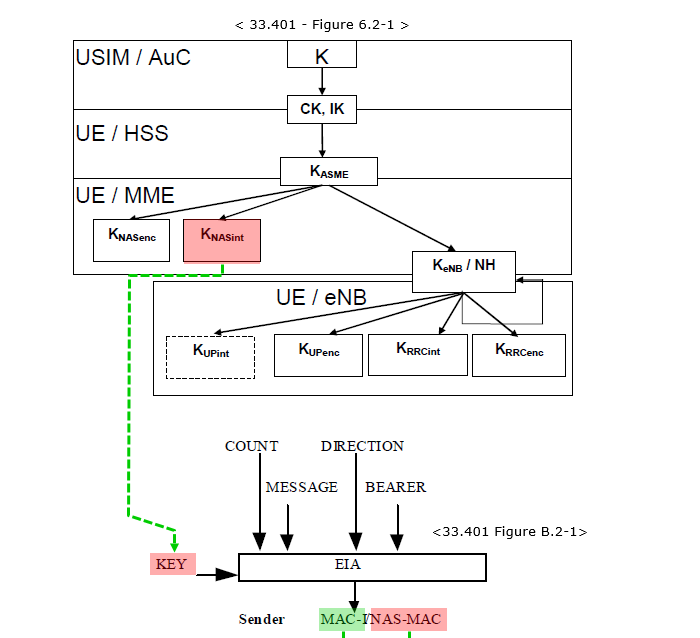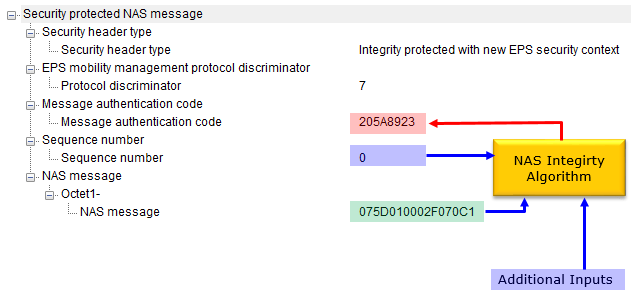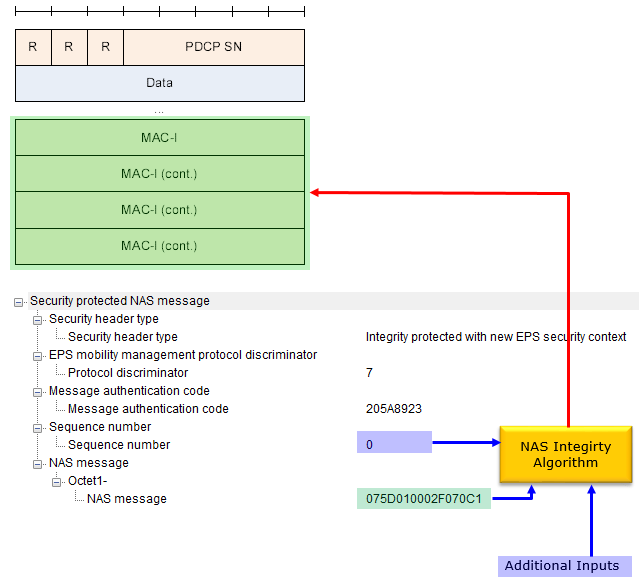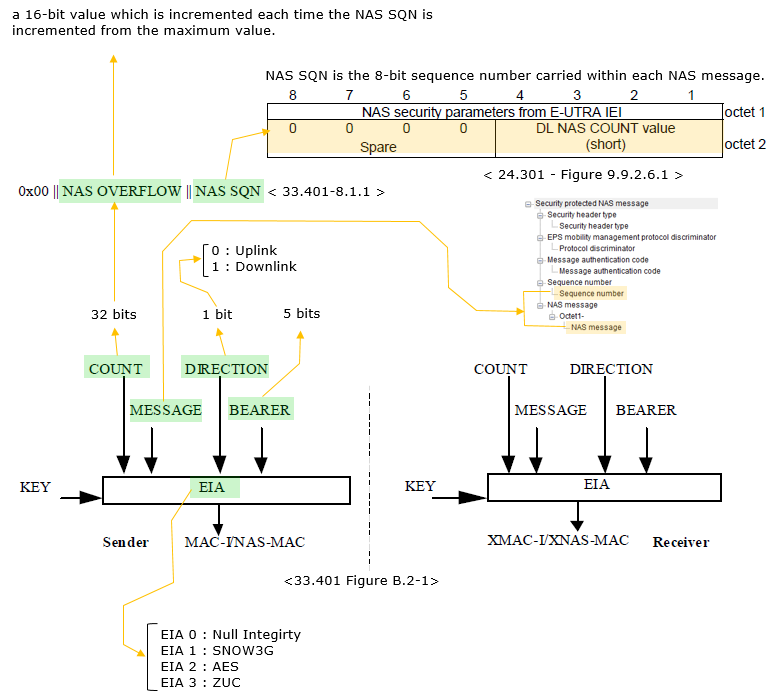LTE Quick Reference NAS Integrity Algorithm   NAS Integrity is a mechanism that can let you (UE or Network) know if a NAS message is modified or corrupted in some way (See Confidentiality and Integrity pagefor the details of the concept of Integrity).   Overall flow from Key Generations and final output for NAS Integrity can be illustrated as follows :Simply put, NAS Integrity (in LTE and UMTS) is to calculate the Message Authentication Code (MAC, shown in Red) using various inputs (shown in Blue and Green) and to calculate MAC-I field in PDCP PDU as illustrated below.The NAS Integrity Algorithm used in LTE is called EIA(EPS Integrity Algorithm). High level functionality of EIA can be illustrated as below.As shown above, there are several different EIA that can be used for LTE and you need to go through a lot of other documents to understand the details of these algorithm.  For EIA1 as an example, refer to SNOW3G page.     Reference :     CryptoMobile (GitHub)